1. /
2. CBSE
3. /
4. Class 12
5. /
6. Mathematics
7. /
8. CBSE Question Paper 2016...

# CBSE Question Paper 2016 class 12 Mathematics### myCBSEguide App

Download the app to get CBSE Sample Papers 2023-24, NCERT Solutions (Revised), Most Important Questions, Previous Year Question Bank, Mock Tests, and Detailed Notes.

CBSE Question Paper 2016 class 12 Mathematics conducted by Central Board of Secondary Education, New Delhi in the month of March 2016. CBSE previous year question papers with solution are available in myCBSEguide mobile app and cbse guide website. The Best CBSE App for students and teachers is myCBSEguide which provides complete study material and practice papers to cbse schools in India and abroad.

CBSE Question Paper 2016 class 12 Mathematics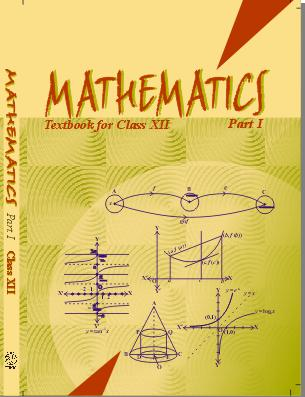## Class 12 Mathematics list of chapters

1. Relations and Functions
2. Inverse Trigonometric Functions
3. Matrices
4. Determinants
5. Continuity and Differentiability
6. Application of Derivatives
7. Integrals
8. Application of Integrals
9. Differential Equations
10. Vector Algebra
11. Three Dimensional Geometry
12. Linear Programming
13. Probability

## CBSE Question Paper 2016 class 12 Mathematics

### General Instruction:

• All question are compulsory.
• Please check that this Question Paper contains 26 Questions.
• Questions 1 to 6 in Section-A are Very Short Answer Type Questions carrying one mark each.
• Questions 7 to 19 in Section-B are Long Answer I Type Questions carrying 4 marks each.
• Questions 20 to 26 in Section-C are Long Answer II Type Questions carrying 6 marks each.
• Please write down the serial number of the Question before attempting it.

### Section A

1. Matrix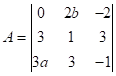is given to be symmetric, find value of a and b.

2. Find the position vector of a point which divides the join of points with position vectors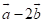and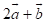externally in the ratio 2:1.

3. The two vectors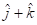and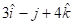represent the two sides AB and AC, respectively of a. Find the length of the median through A.

4. Find the vector equation of plane which is at a distance of 5 units from the origin and its
normal vector is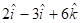.

5. Find the maximum value of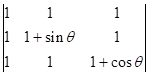6. If A is a square matrix such that A2 = I, then find the simplified value of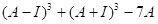### Section B

7. Show that the equation of normal at any point t on the curve x = 3 cost t – cos3t and
y = 3 sin t – sin3t is  4(y cos3t – sin3t) = 3 sin 4t.

8. Find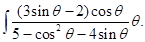### OR

Evaluate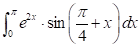9. Find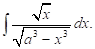10. Evaluate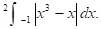11. Find the particular solution of the differential equation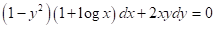Given that y = 0 when x = 1.

12. Find the general solution of the following differential equation: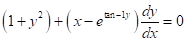13. Show that the vector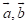and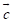are coplanar if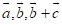and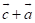are coplanar.

14. Find the vector and Cartesian equations of the line through the point (1, 2, -4) perpendicular to the two lines.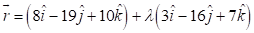and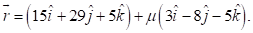15. Three persons A, B and C apply for a job of manager in a Private Company. Chances of their selection (A, B and C) are in the ratio 1:2:4. The probabilities that A, B and C can introduce changes to improve profits of the company are 0.8, 0.5 and 0.3, respectively. If the change does not take place, find the probability that it is due to the appointment of C.

### OR

A and B throw a pair of dice alternately. A wins the game if he gets a total of 7 and B wins the game if he gets a total of 10. If A starts the game, then find the probability that B wins.

These are 15 questions only. To view and download complete question paper with solution install myCBSEguide App from google play store or login to our student dashboard.

## Previous Year Questions Papers for class 12 Maths

Previous Year Questions Papers for class 12 Maths with solution from best CBSE App the myCBSEguide. CBSE class 12 Mathematics question paper 2018 in PDF format with solution will help you to understand the latest question paper pattern and marking scheme of the CBSE board examination. You will get to know the difficulty level of the question paper. CBSE question papers 2016 for class 12 Mathematics have 15 questions with solution.

## CBSE Question Paper 2016

CBSE question papers 2018, 2017, 2016, 2015, 2014, 2013, 2012, 2011, 2010, 209, 2008, 2007, 2006, 2005 and so on for all the subjects are available under this download link. Practicing real question paper certainly helps students to get confidence and improve performance in weak areas.

To download CBSE Question Paper 2016 class 12 Accountancy, Chemistry, Physics, History, Political Science, Economics, Geography, Computer Science, Home Science, Accountancy, Business Studies and Home Science; do check myCBSEguide app or website. myCBSEguide provides sample papers with solution, test papers for chapter-wise practice, NCERT solutions, NCERT Exemplar solutions, quick revision notes for ready reference, CBSE guess papers and CBSE important question papers. Sample Paper all are made available through the best app for CBSE students and myCBSEguide website.### Test Generator

Create question paper PDF and online tests with your own name & logo in minutes.### myCBSEguide

Question Bank, Mock Tests, Exam Papers, NCERT Solutions, Sample Papers, Notes